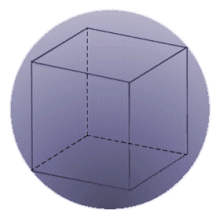# it's pretty. Geometrical probabilityA cube is circumscribed in a sphere. What is the probability that a point (taken randomly) lies inside the sphere, but outside the cube.

If the answer is of the form $(1-\dfrac{1}{\lambda})$ , then what is the value of $\lambda$. (rounded off up to 3 decimal places)

You can try my other Probability problems by clicking here

×

Problem Loading...

Note Loading...

Set Loading...